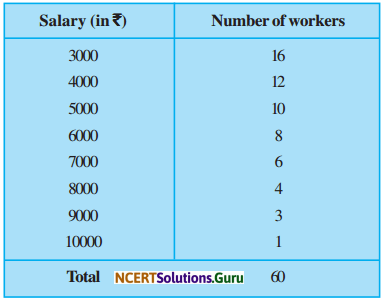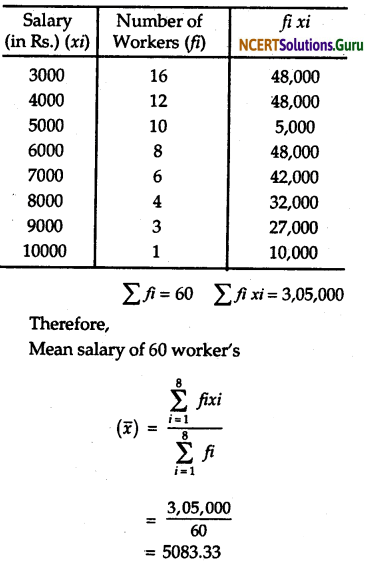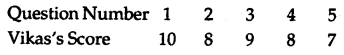# NCERT Solutions for Class 9 Maths Chapter 14 Statistics Ex 14.4

These NCERT Solutions for Class 9 Maths Chapter 14 Statistics Ex 14.4 Questions and Answers are prepared by our highly skilled subject experts.

## NCERT Solutions for Class 9 Maths Chapter 14 Statistics Exercise 14.4

Question 1.
The following number of goals were scored by a team in a series of 10 matches:
2, 3, 4, 5, 0, 3, 3, 4, 3
Find the mean median and mode of these scores.
Solution:
We know thator, Mean = 2.8
To obtain the median we arrange the goals in ascending order to get 0, 1, 2, 3, 3, 4, 4, 5
Since the number of observations is 10.
So, there are two middle terms, i.e. the $$\left(\frac{10^{\mathrm{th}}}{2}\right)$$ and $$\left(\frac{10}{2}+1\right)^{\text {th }}$$ i.e. the 5th and 6th term.
i.e. The median = $$\frac{3+3}{2}$$ = 3
So, the median goat was scored is 3.
In the given observation, 3 occurs most frequently i.e. four times.
So, the mode is 3.Question 2.
In a mathematics test given to 15 students, the following marks (out of 100) are recorded.
41, 39, 48, 52, 46, 62, 54, 40, 96, 52, 98, 40, 42, 52, 60
Find the mean, median and mode of the above marks.
Solution:
We know thatTo obtain the median we arrange the marks in ascending order to get.
39, 40, 40, 41, 42, 46, 48, 52, 52, 54, 54, 60, 62, 96, 98
Since the number of observation is 15.
So their are one middle term i.e. $$\left(\frac{15+1}{2}\right)^{\mathrm{th}}$$ i.e. 8th term.
i.e. the median = 52.
So, the median marks get by students is 52.
In the given observation, 52 occur most frequently i.e. 3 times. So the mode is 52.

Question 3.
The following observations have been arranged in ascending order. If the median of the data is 63, find the value of x.
29, 32, 48, 50, x, x + 2, 72, 78, 84, 95
Solution:
We have given that the data arranged in ascending order i.e. 29, 32, 48, 50, x, x + 2, 72, 78, 84, 95.
Since the number of observations is 10.
So there are two middle terms, i.e. the $$\left(\frac{10^{\mathrm{th}}}{2}\right)$$ and $$\left(\frac{10}{2}+1\right)^{\text {th }}$$ term.
i.e. the 5th and 6th term.
i.e. the median = $$\frac{x+(x+2)}{2}$$
⇒ 63= $$\frac{2 x+2}{2}$$ (∵ median =63)
⇒ 63 = x + 1
⇒ x = 62
Therefore, the value of x is 62.Question 4.
Find the mode of
14, 25, 14, 28, 18, 17, 18, 14, 23, 22, 14, 18
Solution:
we arrange the data in the following form:
14, 14, 14, 14, 17, 18, 18, 18, 22, 23, 25, 28.
Here 14 occur most frequently i.e. four times.
So, the mode is 14.

Question 5.
Find the mean salary of 60 workers of a factory from the following table:Solution:
First, we make the frequency distribution table of given data.Question 6.
Give one example of a situation in which
(i) the mean an appropriate measure of central tendency.
(ii) the mean is not an appropriate measure of central tendency but the median is an appropriate measure of central tendency.
Solution:
Consider a situation when a student Vikas received their test copies.
The test had five questions, each carrying ten marks. His scores were as follows:Vikas’s average score = $$\frac{42}{5}$$ = 8.4
To arranged his scores in ascending order and found out the middle score as:In this situation means is an appropriate measure of central tendency.
(ii) Consider another situation when a student Tanvi received their test copies. The test had again five questions, each carrying the marks. Her scores were as follows:Tanvi’s average score = $$\frac{41}{5}$$ = 8.2
To arranged her scores in ascending order and found out the middle score as:
Tanvi’s Score 4 7 10 10 10
In this situation meaning is not an appropriate measure of central tendency but the median is an appropriate measure of central tendency.

error: Content is protected !!